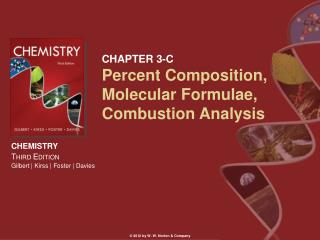DownloadDownload PresentationCHAPTER 3-C Percent Composition, Molecular Formulae, Combustion Analysis

# CHAPTER 3-C Percent Composition, Molecular Formulae, Combustion Analysis

Download Presentation## CHAPTER 3-C Percent Composition, Molecular Formulae, Combustion Analysis

- - - - - - - - - - - - - - - - - - - - - - - - - - - E N D - - - - - - - - - - - - - - - - - - - - - - - - - - -
##### Presentation Transcript

1. CHAPTER 3-C Percent Composition, Molecular Formulae, Combustion Analysis © 2012 by W. W. Norton & Company

2. Percent Composition: Identifies the elements present in a compound as a mass percent of the total compound mass. The mass percent is obtained by dividing the mass of each element by the total mass of a compound and converting to percentage. Percentage Composition

3. Empirical Formula • Theempirical formula gives the ratio of the number of atoms of each element in a compound. CompoundFormulaEmpirical Formula Hydrogen peroxide H2O2 OH Benzene C6H6 CH Ethylene C2H4 CH2 Propane C3H8 C3H8

4. Percentage Composition • Glucose has the molecular formula C6H12O6. What is its empirical formula, and what is the percentage composition of glucose? Empirical Formula = smallest whole number ratio CH2O

5. Percentage Composition CH2O Total mass = 12.01 + 2.02 + 16.00 = 30.03 %C = 12.01/30.03 x 100% = 39.99% %H = 2.02/30.03 x 100% = 6.73% %O = 16.00/30.03 x 100% = 53.28%

6. Percentage Composition Saccharin has the molecular formula C7H5NO3S. What is its empirical formula, and what is the percentage composition of saccharin? Empirical Formula is same as molecular formula MW = 183.19 g/mole %C = (7 x 12.011)/183.19 x 100% = 45.89% etc.

7. A compound’s empirical formula can be determined from its percent composition. A compound’s molecular formula is determined from the molar mass and empirical formula. Empirical Formula

8. A compound was analyzed to be 82.67% carbon and 17.33% hydrogen by mass. What is the empirical formula for the compound? Assume 100 g of sample, then 82.67 g are C and 17.33 g are H Empirical Formula

9. Convert masses to moles: 82.67 g C x mole/12.011 g = 6.88 moles C 17.33 g H x mole/1.008 g = 17.19 mole H Find relative # of moles (divide by smallest number) Empirical Formula

10. Convert moles to ratios: 6.88/6.88 = 1 C 17.19/6.88 = 2.50 H Or 2 carbons for every 5 hydrogens C2H5 Empirical Formula

11. Empirical Formula is: C2H5 Formula weight is: 29.06 g/mole If the molecular weight is known to be 58.12 g/mole Then the molecular formula must be: C4H10 Empirical Formula

12. Empirical Formula • Combustion analysis is one of the most common methods for determining empirical formulas. • A weighed compound is burned in oxygen and its products analyzed by a gas chromatogram. • It is particularly useful for analysis of hydrocarbons.

13. Combustion Analysis Combustion Analysis: the technique of finding the mass composition of an unknown sample (X) by examining the products of its combustion. X + O2 → CO2 + H2O 0.250 g of compound X produces: 0.686 g CO2 and 0.562 g H2O

14. Combustion Analysis X + O2 → CO2 + H2O 1. Find the mass of C & H that must have been present in X (multiply masses of products by percent composition of the products). C: 0.686 g x 12.01 g/44.01 g = 0.187 g C H: 0.562 g x (2 x 1.008 g)/18.02 g = 0.063 g H

15. Combustion Analysis X + O2 → CO2 + H2O 0.187 g C + 0.063 g H = 0.250 g total So compound X must contain only C and H!! 2. Find the number of moles of C and H C: 0.187 g x mole/12.01 g = 0.0156 moles C H: 0.063 g x mole/1.008 g = 0.063 moles H

16. Combustion Analysis X + O2 → CO2 + H2O 3. Find the RELATIVE number of moles of C and H in whole number units (divide by smallest number of moles) C: 0.0156/0.0156 = 1 H: 0.063/0.0156 = 4 If these numbers are fractions, multiply each by the same whole number.

17. Combustion Analysis X + O2 → CO2 + H2O 3. Write the Empirical Formula (use the relative numbers as subscripts) CH4

18. Combustion Analysis Summary • Find the mass of C and H in the sample. • Find the actual number of moles of C and H in the sample. • Find the relative number of moles of C and H in whole numbers. • Write the empirical formula for the unknown compound.

19. Combustion Analysis NOTE! In step # 1 always check to see if the total mass of C and H adds up to the total mass of X combusted. If the combined mass of C and H is less than the mass of X, then the remainder is an unknown element (unless instructed otherwise). If a third element is known, calculate the mass of that element by subtraction (at the end of step 1), and include the element in the remaining steps.

20. Combustion Analysis Combustion Analysis provides the Empirical Formula. If a second technique provides the molecular weight, then the molecular formula may be deduced. • Calculate the empirical formula weight. • Find the number of “formula units” by dividing the known molecular weight by the formula weight. • Multiply the number of atoms in the empirical formula by the number of formula units.

21. Combustion Analysis The molecular weight of glucose is 180 g/mole and its empirical formula is CH2O. Deduce the molecular formula. • Formula weight for CH2O is 30.03 g/mole • # of formula units = 180/30.03 = 6 • Molecular formula = C6H12O6